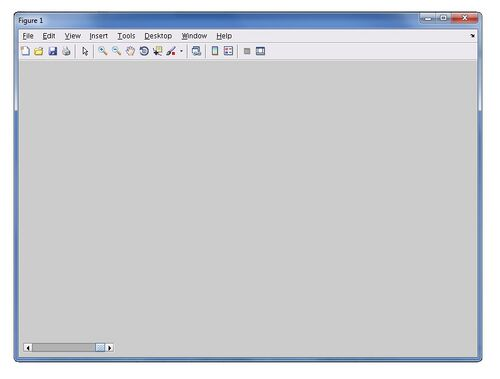# MATLAB Tutorial 2

## Using FVG Sheet Vec

This example demonstrates how to plot vectors of current velocity as a field of arrows in matlab. This is done using fvg_sheet_vec which is a similar function to fvg_sheet.

## Basic Setup

Just as with tutorial 1, an fvgraphics object must first be created, then some axes need to created and adjusted.

```fobj = fvgraphics;

ax = gca;

axis(ax,'equal')
axis(ax,'off')
mycolor(ax,[0 0.5 20])     % BMT function for customized colorbars
```Note: You cannot see the axis as we have made it invisible already. But it's there!

Now it's time to plot the vectors using fvg_sheetvec. The variables that can be plotted are only the variables with x and y components, current velocity, wind speed, wave direction, wave shear, and sediment load.

```modfil = 'C:/users/COM/TUFLOWFV/results/example_model_output.nc';

vecObj = fvg_sheetvec(fobj,modfil,'variables','V','peerObj',ax,'TitleTime','on')
```

With just the vectors there it is a little hard to discern anything useful. We can plot a normal fvg_sheet on the same axis.

```sheetObj = fvg_sheet(fobj,modfil,'variables','V','peerObj',ax)
```

Much of the functionality of fvg_sheetvec is actually similar to fvg_sheet. You can examine the editable properties in MATLAB to experiment with different settings. Lets make the spacing of the vectors smaller and increase their size a little bit.

```set(vecObj,'vecgrid',15,'vecscale',100)
```

#### A Note on Vector Grids

fvg_sheetvec plots arrows (or possibly cones with a setting change) on a pixel grid. This grid is interpolated to from the model mesh. The advantage of a pixel grid is it allows scale and spacing to be easily customized, and to automatically update with zooming. The settings above specified that the property vecgrid be a grid of 15px spacing and the vecscale be a scale where a vector of magnitude 1 (in this case m/s) is 100px long.

## Default Vectors in FVG Sheet

Similar basic vectors can be created straight from an fvg sheet object. FVG sheet objects have a property 'vectors' which, when set to 'on', creates an fvg sheet vec object and places the handle as a property of the fvg sheet. The disadvantage of using such a system is that you are limited to variables stored within the same resfil as the fvg sheet (you may have seperate output files for currents than say, sediments) and the object is not as intuitively customizable. In many cases if a user wants customizable, report quality vector figures, then it is recommended that they use fvg sheet vec. If however, you just want to see simple velocity vectors on top of your fvg sheet, switch vectors to on.